﻿ Functional Influence Evaluation of Copper Addition and Pb-Sb-Cu Alloy Melting Temperature on the Alloy Electrical Conductivity

### Functional Influence Evaluation of Copper Addition and Pb-Sb-Cu Alloy Melting Temperature on the All...

C. I. Nwoye, S. O. Nwakpa, V. U. Nwoke, D. D. Abubakar, C. C. Nwangwu, I. Obuekwe

## Functional Influence Evaluation of Copper Addition and Pb-Sb-Cu Alloy Melting Temperature on the Alloy Electrical Conductivity

C. I. Nwoye1,, S. O. Nwakpa1, V. U. Nwoke1, D. D. Abubakar1, 2, C. C. Nwangwu1, I. Obuekwe1, 3

1Department of Metallurgical and Materials Engineering, Nnamdi Azikiwe University, Awka, Nigeria

2Ajaokuta Steel Company, Kogi State, Nigeria

3Scientific Equipment Development Institute, Enugu, Nigeria

### Abstract

Studies were carried out to evaluate the functional influence of copper addition and Pb-Sb-Cu alloy melting temperature on the alloy electrical conductivity. The alloy was cast by pouring a stirred mixture of heated Pb-Sb alloy and powdered copper into a sand mould and then furnace cooled. Results of electrical test carried out indicate that the electrical conductivity of the Pb-Sb-Cu alloy increases with increase in the melting temperature of the Pb-Sb-Cu alloy. This invariably implied decrease in the electrical resistance and resistivity of the alloy with increase in the melting temperature, in accordance with findings that the minimum additional energy (energy gap) which a bonding electron must acquire to conduct electricity, decreases with decrease in the electrical resistance, resistivity and with increasing temperature. Increased copper addition (5- 45g) to the base alloy (Pb-Sb) was discovered to increase correspondingly the electrical conductivity. This is attributed to the increased melting temperature of the alloy as a result of increased impurity atoms in the alloys in the form of copper. In order to complement the experimental result, a model was derived and used as a tool for evaluating the functional influence of the two process parameters; copper input and alloy melting temperature on the electrical conductivity of Pb-Sb-Cu alloy. The derived model is expressed as; α = 0.0074 ɤ2 - 0.0031ɤ + 0.0325 T2 – 26.9945 T + 5693.357 The validity of the two-factorial model was found to be rooted on the expression 1.756 x 10-4 α - 1= 1.3 x 10-6 ɤ2 + 5.71 x 10-6 T2 – 5.44 x 10-7ɤ – 4.74 x10-3 T where both sides of the expression are correspondingly approximately equal. Statistical analysis of the derived model-predicted, regression model-predicted and experimental results for each value of copper mass-input and alloy melting temperature considered shows standard errors of 3.0470, 0.0002 & 4.3231% and 2.7140, 0.0004 & 2.2943% respectively. Furthermore, electrical conductivity per unit copper mass-input as obtained from derived model-predicted, regression model-predicted and experimental results are 0.7862, 0.7025 and 0.835 (Ωm)-1 g-1 respectively. Similarly, electrical conductivity per unit rise in the alloy melting temperature as obtained from derived model-predicted, regression model-predicted and experimental results are 2.0966, 2.2086 and 2.2267 (Ωm)-1 / 0C respectively. Deviational analysis indicates that the maximum deviation of derived model-predicted electrical conductivity from experimental results is less than 4%; implying over 96% viable model operational confidence level.

• C. I. Nwoye, S. O. Nwakpa, V. U. Nwoke, D. D. Abubakar, C. C. Nwangwu, I. Obuekwe. Functional Influence Evaluation of Copper Addition and Pb-Sb-Cu Alloy Melting Temperature on the Alloy Electrical Conductivity. International Journal of Materials Lifetime. Vol. 2, No. 1, 2015, pp 30-37. http://pubs.sciepub.com/ijml/2/1/5
• Nwoye, C. I., et al. "Functional Influence Evaluation of Copper Addition and Pb-Sb-Cu Alloy Melting Temperature on the Alloy Electrical Conductivity." International Journal of Materials Lifetime 2.1 (2015): 30-37.
• Nwoye, C. I. , Nwakpa, S. O. , Nwoke, V. U. , Abubakar, D. D. , Nwangwu, C. C. , & Obuekwe, I. (2015). Functional Influence Evaluation of Copper Addition and Pb-Sb-Cu Alloy Melting Temperature on the Alloy Electrical Conductivity. International Journal of Materials Lifetime, 2(1), 30-37.
• Nwoye, C. I., S. O. Nwakpa, V. U. Nwoke, D. D. Abubakar, C. C. Nwangwu, and I. Obuekwe. "Functional Influence Evaluation of Copper Addition and Pb-Sb-Cu Alloy Melting Temperature on the Alloy Electrical Conductivity." International Journal of Materials Lifetime 2, no. 1 (2015): 30-37.

 Import into BibTeX Import into EndNote Import into RefMan Import into RefWorks

12345
Prev Next

### 1. Introduction

Studies [1, 2, 3] have been carried out to improve the electrical conductivity of Pb-Sb alloy used as wet cell battery heads. Blumenthal  discovered that addition of cadmium enhances the electrical conductivity of Pb-Sb alloy tremendously. It was however, stated that the alloy cannot find application in battery heads and plates because Cd is very radioactive and causes a volatile and explosive reaction when in contact with sulphuric acid for a long time.

Rollason and Hysel  reported that addition of silver to Pb-Sb alloy increases very significantly the electrical conductivity of the alloy. It was however, stated that this increase does not give a stable value due to impurities in the Ag. It was stated that these impurities are Au, As, Sn, Cu and S. He further posited that these impurities create an unstable electrical field in the alloy of Pb-Sb-Ag. It is believed that this short coming has made the use of this alloy for battery heads and plates impossible since it obscures the precise electromotive force of the electrolyte in the battery.

Nwoye  found that addition of copper powder by dispersion to Pb-Sb alloy improves the electrical conductivity of alloy greatly. It is believed that this breakthrough was possible because Cu used, had high purity level (99.8%).

It has been reported  that the higher the temperature applied to metals and alloys, the greater the bonds broken, and the higher the level of conductivity attained. The same report indicates that the resistivity of engineering materials such as metals and alloys is dependent on the temperature at which the material is exposed. The report  concluded that increase in temperature of materials reduces its resistivity. Ijomah  reported that the energy gap; minimum additional energy which a bonding electron must acquire to leave the bond and, hence become free to conduct electricity decreases with increasing temperature and impurity content. The report also revealed that the resistivity increases with increase in the energy gap.

The aim of this work is to evaluate the functional influence of copper addition and the alloy melting temperature on its (alloy) electrical conductivity.

### 2. Materials and Methods

Alloy preparation

The materials used are antimonial lead scraps and electrolytic copper powder of grain size < 425μm. The antimonial lead collected were melted together in order to obtain a fairly uniform composition of lead antimonial alloy, in case of any variation in antimony content. The melting operation was carried out at the forge, followed by casting of the alloys in sand mould and cutting to various sizes for use in the actual alloying. They melting crucible was of 260mm long, 200mm wide mild steel of about 100mm breadth with handle for carriage.

Mould preparation

The preparation of the mould was done by first sieving the sand for aeration and mixing 6% moisture to give good green strength. The mould box of dimension 300mm wide, 100mm breadth and 500mm long was made from cast metal frame. A long hollow cylindrical pipe of 85mm long and 9mm diameter was used as the pattern for the cast. The mould was allowed to dry.

Casting and cooling

A weighed quantity of lead antimony alloy (500g) was placed on the crucible and then placed inside the furnace. The alloy was cast by pouring a stirred mixture of Cu powder and Pb-Sb alloy heated to 420°C, into a sand mould and then were cooled in the furnace. The Control alloys were cast by just pouring only the molten Pb-Sb into the mould.

Heat treatment

The cast alloys were cut to lengths: 0.075m before being heat treated at a temperature of 1800C for 1 hr to relieve stresses incurred during solidification of the alloys. The heat treatment was carried out to also homogenize the microstructure of the alloys prior to the impact testing process.

Electrical measurements

Following the heat treatment process, electric current I, flowing through the alloy was measured and other electrical properties associated with current flow calculated using appropriate equations. The electromotive force from the dry battery equals 2.9V, being voltage supply to the alloys. The tested specimen were thereafter melted and their melting temperatures recorded correspondingly against their respective values of electric current, resistance, resistivity and conductivity.

Calculation of electrical parameters

According to Ohm’s law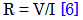(1)

Where

R = Resistance of the alloy (Ω)

V = Voltage supply to the alloy (V)

I = Current flowing through the alloy (A).

The resistance of the alloy was calculated using equation (1). Also, the resistivity of the alloy was calculated using the equation;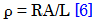(2)

Where

ρ = Resistivity of the alloy (Ωm)

L = Length of the alloy material (m)

A = Cross sectional area of the alloy (m2).

The cross sectional area of the alloy material was calculated using the equation;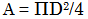(3)

Where

Π = 22/7 Substituting these values into

D = 0.9cm; (Diameter of cross- section of the sample).

Substituting these values into equation (3)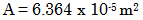Also, the conductivity of the alloy was calculated as the reciprocal of the resistivity;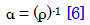(4)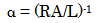(5)

Where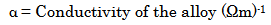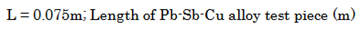2.1. Model Formulation

Experimental data obtained from the highlighted research work were used for the model derivation. Computational analysis of these data shown in Table 2, gave rise to Table 3 which indicate that;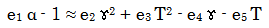(6)

Introducing the values of the empirical constants e1, e2, e3, e4, and e5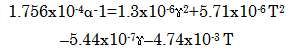(7)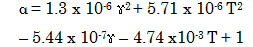(8)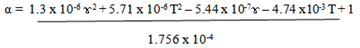(9)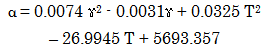(10)

Where

(α) = Electrical conductivity (Ωm)-1

(ɤ) = Mass-input of copper powder (g)

T = Melting temperature of Pb-Sb-Cu alloy (0C)

e1, e2, e3, e4, and e5 = Empirical constants (determined using C-NIKBRAN )

e1 = 1.756 x 10-4 , e2 = 1.3 x 10-6 , e3 = 5.71 x 10-6 , e4 = 5.44 x 10-7 and e5 = 4.74 x 10-3

2.2. Boundary and Initial Condition

Consider Pb-Sb alloy (in a furnace) garnished with copper powder. The furnace atmosphere is not contaminated i.e (free of unwanted gases and dusts). Initially, atmospheric levels of oxygen are assumed just before adding copper to Pb-Sb alloy (due to air in the furnace). Mass of Pb-Sb alloy used in each case: 500g, length of cast alloys: 0.075m, Pb-Sb-Cu alloy melting temperature range: 425- 440°C, heat treatment temperature: 180°C.

The boundary conditions are: furnace oxygen atmosphere at the top and bottom of the melt interacting with the gas phase. At the bottom of the particles, a zero gradient for the gas scalar are assumed and also for the gas phase at the top of the particles. The sides of the particles are taken to be symmetries.

### 3. Results and Discussions

Results of chemical analysis carried out on the materials used (as shown in Table 1) indicate that antimonial lead contains about 3.3% Cu in addition to Pb and Sb present. The percentage composition of the powdered Cu used is as received.

#### Table 1. Chemical composition of materials usedDownload asVeiw tableTables index

#### Table 2. Electrical properties of Pb-Sb alloy cooled in furnace (Alloy control of melting temperature 425°C)Download asVeiw tableTables index

Table 2 shows the electrical properties of furnace cooled Pb-Sb alloy (control). This alloy melted at a temperature of 425°C.

Increasing the temperature of metals and alloys reduces their respective resistivities . Therefore, substituting equation (1) into (2) gives ρ = V A/L I which shows an inverse relationship between current I, and resistivity, ρ. This indicates (as shown in Table 3) that decrease in the resistivity of the alloys result to increase in the current flowing through the alloy. This is sequel to the fact that increase in the temperature of metals and alloys excites the electron and increases the number bonds broken resulting to increased flow of electron (through increased vibrational motion) into the conduction band .

#### Table 3. Effect of copper addition (to Pb-Sb alloy) and melting temperature of Pb-Sb-Cu alloy on the electrical current flow, resistance, resistivity and conductivity of the Pb-Sb-Cu alloy formedDownload asVeiw tableTables index

Furthermore, the minimum additional energy (energy gap) which a bonding electron must acquire to leave the bond in the valence band and move into the conduction band hence, becoming free to conduct electricity, decreases with increasing temperature and invariably decreasing electrical resistance and resistivity. The electrical conductivity α of the Pb-Sb-Cu alloy is calculated from equations (3) and (4) as the reciprocal of the electrical resistivity ρ. Table 3 also shows that increase in copper addition (up to 45g) to the base alloy (Pb-Sb) increases correspondingly the current flow, electrical conductivity and decreased correspondingly the electrical resistance and resistivity of Pb-Sb-Cu alloy so produced. This is because Cu added to the base alloy (Pb-Sb) behaves like impurity atoms which have been reported  to reduce the electrical resistivity of the alloys involved. It is therefore expected that increased Cu addition into the base alloy will also result in much reduction in the resistivity of the alloy and hence much increase in the current flow. This is attributed to the increased melting temperature of the alloy as a result of increased impurity atoms in the alloys in the form of copper.

3.1. Model Validation

The validity of the model is strongly rooted in the core model equation (equation (7)) where both sides of the equation are correspondingly approximately equal. Table 4 also agrees with equation (7) following the values of 1.756 x 10-4 α - 1= 1.3 x 10-6 ɤ2 + 5.71 x 10-6 T2 – 5.44 x 10-7ɤ – 4.74 x10-3 Tevaluated from the experimental results in Table 3.

#### Table 4. Variation of 1.756 x 10-4 α – 1 with 1.3 x 10-6 ɤ2 + 5.71 x 10-6T2 – 5.44 x 10-7ɤ – 4.74 x10-3 TDownload asVeiw tableTables index

Furthermore, the derived model was validated by comparing the electrical conductivity predicted by the model and that obtained from the experiment. This was done using various evaluative techniques such as computational, statistical, graphical and deviational analysis.

Computational Analysis

Computational analysis of the experimental and model-predicted limit of desulphurization was carried out to ascertain the degree of validity of the derived model. This was done by comparing limits of desulphurization per unit rise in treatment temperature and per unit mass-input of KClO3 evaluated from model-predicted results with those from actual experimental results

Electrical conductivity per unit copper mass-input (Ωm)-1 g-1 was calculated from the equation;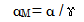(11)

Therefore, a plot of the electrical conductivity against copper mass-input as in Figure 1 using experimental results in Table 2, gives a slope, S at points (5, 87.7) and (45, 121.1) following their substitution into the mathematical expression;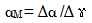(12)

Equation (12) is detailed as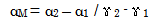(13)

Where

Δα = Change in electrical conductivity of α 2 , α 1 at two copper mass-input values ɤ 2, ɤ 1. Considering the points(5, 87.7) and (45, 121.1) for (ɤ1, α 1) and (ɤ 2, α 2) respectively, and substituting them into equation (13), gives the slope as 0.835 (Ωm)-1 g-1 which is the electrical conductivity per unit copper mass-input during the actual current flow process.Download asVeiw figureFigures index
Figure 1. Coefficient of determination between electrical conductivity and copper mass-input as obtained from the experimentDownload asVeiw figureFigures index
Figure 2. Coefficient of determination between electrical conductivity and copper mass-input as obtained from derived model

A plot of the electrical conductivity against copper mass-input (as in Figure 2) using derived model-predicted results gives a slope: 0.7862 (Ωm)-1 g-1 on substituting the points (5, 91.174) and (45,122.623) for (ɤ1, α1) and (ɤ2, α2) respectively into equation (13). This is the model-predicted electrical conductivity per unit copper mass-input.

Similarly, a plot of the electrical conductivity against copper mass-input (as in Figure 3) using regression model-predicted results gives a slope: 0.7025 (Ωm)-1 g-1 on substituting the points (5, 88.556) and (45, 116.654) for (ɤ1, α 1) and (ɤ 2, α 2) respectively into equation (13). This is the regression model-predicted electrical conductivity per unit copper mass-input. The regression model prediction was carried out relative to the copper mass-input.Download asVeiw figureFigures index
Figure 3. Coefficient of determination between electrical conductivity and copper mass-input as obtained from regression model

Electrical conductivity per unit rise in alloy melting temperature (Ωm)-1 /0C was calculated from the equation;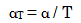(14)Download asVeiw figureFigures index
Figure 4. Coefficient of determination between electrical conductivity and alloy melting temperature as obtained from the experiment

Therefore, a plot of the electrical conductivity against alloy melting temperature as in Figure 4 using experimental results in Table 2, gives a slope, S = 2.2267 (Ωm)-1 /0C, at points (425, 87.7) and (440, 121.1) for (ɤ1, α 1) and (ɤ 2, α 2) respectively, following their substitution into the mathematical expression;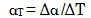(15)

detailed as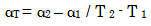(16)

Where

Δα = Change in electrical conductivity of α 2, α 1 at two melting temperature values T2, T 1. This is the electrical conductivity per unit rise in alloy melting temperature during the actual current flow process.Download asVeiw figureFigures index
Figure 5. Coefficient of determination between electrical conductivity and alloy melting temperature as obtained from the experiment

A plot of the electrical conductivity against alloy melting temperature (as in Figure 5) using derived model-predicted results gives a slope: 2.0966 (Ωm)-1 /0C on substituting the points (425, 91.174) and (440, 122.623) for (ɤ1, α 1) and (ɤ 2, α 2) respectively into equation (16). This is the model-predicted electrical conductivity per unit rise in alloy melting temperature.

Furthermore, a similarly plot of the electrical conductivity against alloy melting temperature (as in Figure 6) using regression model-predicted results gives a slope: 2.2086 (Ωm)-1 /0C on substituting the points (425, 85.672) and (440, 118.801) for (ɤ1, α 1) and (ɤ 2, α 2) respectively into equation (16). This is the regression model-predicted electrical conductivity per unit rise in alloy melting temperature. Here, the regression model prediction was carried out relative to the Pb-Sb-Cu alloy melting temperature.Download asVeiw figureFigures index
Figure 6. Coefficient of determination between electrical conductivity and alloy melting temperature as obtained from regression model

A comparison of these three sets of values for electrical conductivity (per unit copper mass-input and per unit rise in alloy melting temperature also shows proximate agreement and a high degree of validity of the derived model.

Statistical Analysis

Standard Error

The standard error (STEYX) in predicting electrical conductivity (using results from derived model, regression model and experiment) for each value of the copper mass-input and alloy melting temperature are indicated in Table 5. The standard error was evaluated using Microsoft Excel version 2003.

#### Table 5. Comparison of the standard errors (%) evaluated from D-Model, R-Model predicted and ExD, results based on copper mass-input and alloy melting temperatureDownload asVeiw tableTables index

Correlation

The correlations between electrical conductivity and copper mass-input as well as electrical conductivity and alloy melting temperature as obtained from derived model, regression model and experiment considering the coefficient of determination R2 from Figure 1-Figure 6 was calculated using Microsoft Excel version 2003.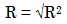(17)

The evaluations correlations are shown in Table 6. These evaluated results indicate that the derived model predictions are significantly reliable and hence valid considering its proximate agreement with results from regression model and actual experiment.

#### Table 6. Comparison of the correlation evaluated from D-Model, R-Model predicted and ExD results based on copper mass-input and alloy melting temperatureDownload asVeiw tableTables index

Graphical Analysis

Comparative graphical analysis of Figure 7 and Figure 8 shows very close alignment of the curves from model-predicted electrical conductivity (MoD) and that of the experiment (ExD). The degree of alignment of these curves is indicative of the proximate agreement between both experimental and model-predicted electrical conductivity.Download asVeiw figureFigures index
Figure 7. Comparison of the electrical conductivities (relative to copper mass-input) as obtained from experiment and derived modelDownload asVeiw figureFigures index
Figure 8. Comparison of the electrical conductivities (relative to alloy melting temperature) as obtained from experiment and derived model

Comparison of derived model with standard model (from Microsoft Excel)

The validity of the derived model was further verified through application of the regression model (Least Square Method (ReG)) in predicting the trend of the experimental results. Comparative analysis of Figure 9 and Figure 10 shows very close alignment of curves and significantly similar trend of data point’s distribution for experimental (ExD), derived model-predicted (MoD) and regression model predicted (ReG) results of electrical conductivity.Download asVeiw figureFigures index
Figure 9. Comparison of the electrical conductivities (relative to copper mass-input) as obtained from experiment, derived model and regression modelDownload asVeiw figureFigures index
Figure 10. Comparison of the electrical conductivities (relative to alloy melting temperature) as obtained from experiment, derived model and regression model

Deviational Analysis

Comparative analysis of electrical conductivity from the experiment and derived model revealed deviations on the part of the model-predicted values relative to values obtained from the experiment. This is attributed to the fact that the surface properties of the Pb-Sb-Cu melt and the physiochemical interactions between the Pb-Sb and the copper powder which were found to have played vital roles during the process were not considered during the model formulation. This necessitated the introduction of correction factor, to bring the model-predicted electrical conductivity to those of the corresponding experimental values.

Deviation (Dn) of model-predicted electrical conductivity from that of the experiment is given by.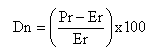(18)

Where

Pr = Electrical conductivity as predicted by derived model ((Ωm))

Er = Electrical conductivity as obtained from experiment ((Ωm))

Correction factor (Cr ) is the negative of the deviation i.e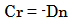(19)

Therefore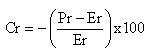(20)

Introduction of the corresponding values of Cr from equation (20) into the derived model gives exactly the electrical conductivity as obtained from experiment.Download asVeiw figureFigures index
Figure 11. Variation of model-predicted electrical conductivity with associated deviation from experimental results (relative to copper mass-input)

Deviational analysis (from Figure 11 and Figure 12) indicates that the maximum deviation of derived model-predicted electrical conductivity from experimental results is less than 4% implying over 96%; a viable model operational confidence. Furthermore, Figure 11 and Figure 12 show that the least and highest deviations of model-predicted electrical conductivity are -1.26 and -3.96%. This corresponds to electrical conductivities: 122.623 and 91.174 (Ωm)-1, alloy melting temperature: 440 and 4250C and copper mass-input: 45 and 45g. This implies that the derived model can exclusive be significantly and viably operational within these process conditions.Download asVeiw figureFigures index
Figure 12. Variation of model-predicted electrical conductivity with associated deviation from experimental results (relative to alloy melting temperature)Download asVeiw figureFigures index
Figure 13. Variation of model-predicted electrical conductivity with associated correction factor (relative to copper mass-input)

Comparative analysis of Figure 11 -Figure 14 indicates that the orientation of the curve in Figure 13 and Figure 14 is opposite that of the deviation of model-predicted electrical conductivity (Figure 11 and Figure 12). This is because correction factor is the negative of the deviation as shown in equations (19) and (20).

Correction factor (from Figure 13 and Figure 14) indicates that least and highest correction factors to the model-predicted electrical conductivity are + 1.26 and + 3.96%. This also corresponds to electrical conductivities: 122.623 and 91.174 (Ωm)-1, alloy melting temperature: 440 and 4250C and copper mass-input: 45 and 45g.Download asVeiw figureFigures index
Figure 14. Variation of model-predicted electrical conductivity with associated correction factor (relative to alloy melting temperature)

It is believed that the correction factor takes care of the effects of surface properties of the Pb-Sb-Cu melt and the physiochemical interactions between the Pb-Sb and the copper powder which were found to have played vital roles during the process were not considered during the model formulation.

It is important to state that the deviation of model predicted results from that of the experiment is just the magnitude of the value. The associated sign preceding the value signifies that the deviation is a deficit (negative sign) or surplus (positive sign).

### 4. Conclusions

The functional influence of copper addition and Pb-Sb-Cu alloy melting temperature on the alloy electrical conductivity has been evaluated. Results of electrical test carried out indicate that the electrical conductivity of the Pb-Sb-Cu alloy increases with increase in the melting temperature of the Pb-Sb-Cu alloy. This implied decrease in the electrical resistance and resistivity of the alloy with increase in the melting temperature, in accordance with findings that the minimum additional energy (energy gap) which a bonding electron must acquire to conduct electricity, decreases with decrease in the electrical resistance, resistivity and with increasing temperature. Increased copper addition (5- 45g) to the base alloy (Pb-Sb) was discovered to increase correspondingly the electrical conductivity. This is attributed to the increased melting temperature of the alloy as a result of increased impurity atoms in the alloys in the form of copper. The validity of the two-factorial model derived and used as a tool for evaluating the functional influence of the two process parameters; copper input and alloy melting temperature, on the electrical conductivity of Pb-Sb-Cu was found to be rooted on the expression 1.756 x 10-4 α - 1= 1.3 x 10-6 ɤ2 + 5.71 x 10-6 T2 – 5.44 x 10-7ɤ – 4.74 x10-3 T where both sides of the expression are correspondingly approximately equal. Statistical analysis of the derived model-predicted, regression model-predicted and experimental results for each value of copper mass-input and alloy melting temperature considered shows standard errors of 3.0470, 0.0002 & 4.3231% and 2.7140, 0.0004 & 2.2943% respectively. Furthermore, electrical conductivity per unit copper mass-input as obtained from derived model-predicted, regression model-predicted and experimental results are 0.7862, 0.7025 and 0.835 (Ωm)-1 g-1 respectively. Similarly, electrical conductivity per unit rise in the alloy melting temperature as obtained from derived model-predicted, regression model-predicted and experimental results are 2.0966, 2.2086 and 2.2267 (Ωm)-1 / 0C respectively. Deviational analysis indicates that the maximum deviation of derived model-predicted electrical conductivity from experimental results is less than 4%; implying over 96% viable model operational confidence level.

### References

  Blumenthal B. (1944). Effects of Silver on the Electrical Conductivity of Lead-Antimony Alloy, Trans. Amer. Inst. Min. Met, England. 149-156.In article  Rollason, E. C., and Hysel, V. B. (1940). Effect of Cadmium on the Electrical Conductivity of Lead-Antimony Alloy, J. Inst. Metals, London. 59-66.In article  Nwoye, C. I. (2000). Effect of Copper Powder Dispersion on the Electrical Conductivity of Lead-Antimony Alloy, M. Eng. Thesis, Nnamdi Azikiwe University, Awka.In article  Nwoye, C. I. (2008). C-NIKBRAN; Data Analytical Memory.In article  Ijomah, M. N. C. (1992).The Structure and Properties of Engineering Materials, Christon Publishers, Awka. pp 56-58.In article  Okeke, P. C. (1987). Introduction to Physics, Gerek Publishers, Enugu. pp 85-93.In article